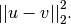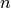# scipy.spatial.distance.sqeuclidean¶

scipy.spatial.distance.sqeuclidean(u, v)

Computes the squared Euclidean distance between two n-vectors u and v, which is defined asParameters : u : ndarray An-dimensional vector. v : ndarray An-dimensional vector. d : double The squared Euclidean distance between vectors u and v.

#### Previous topic

scipy.spatial.distance.sokalsneath

#### Next topic

scipy.spatial.distance.yule